# Visualizing Perimeter and Area Algebraically – Pool Noodles

## Math Task Redesign: Creating Visuals and Adding Context

### Problem: Finding the Perimeter and Area of Composite Figures With An Unknown

In both the MFM1P Grade 9 Applied and MPM1D Grade 9 Academic Ontario math courses, students are required to find the perimeter and area of composite figures given dimensions involving an unknown value. A typical textbook problem looks like this: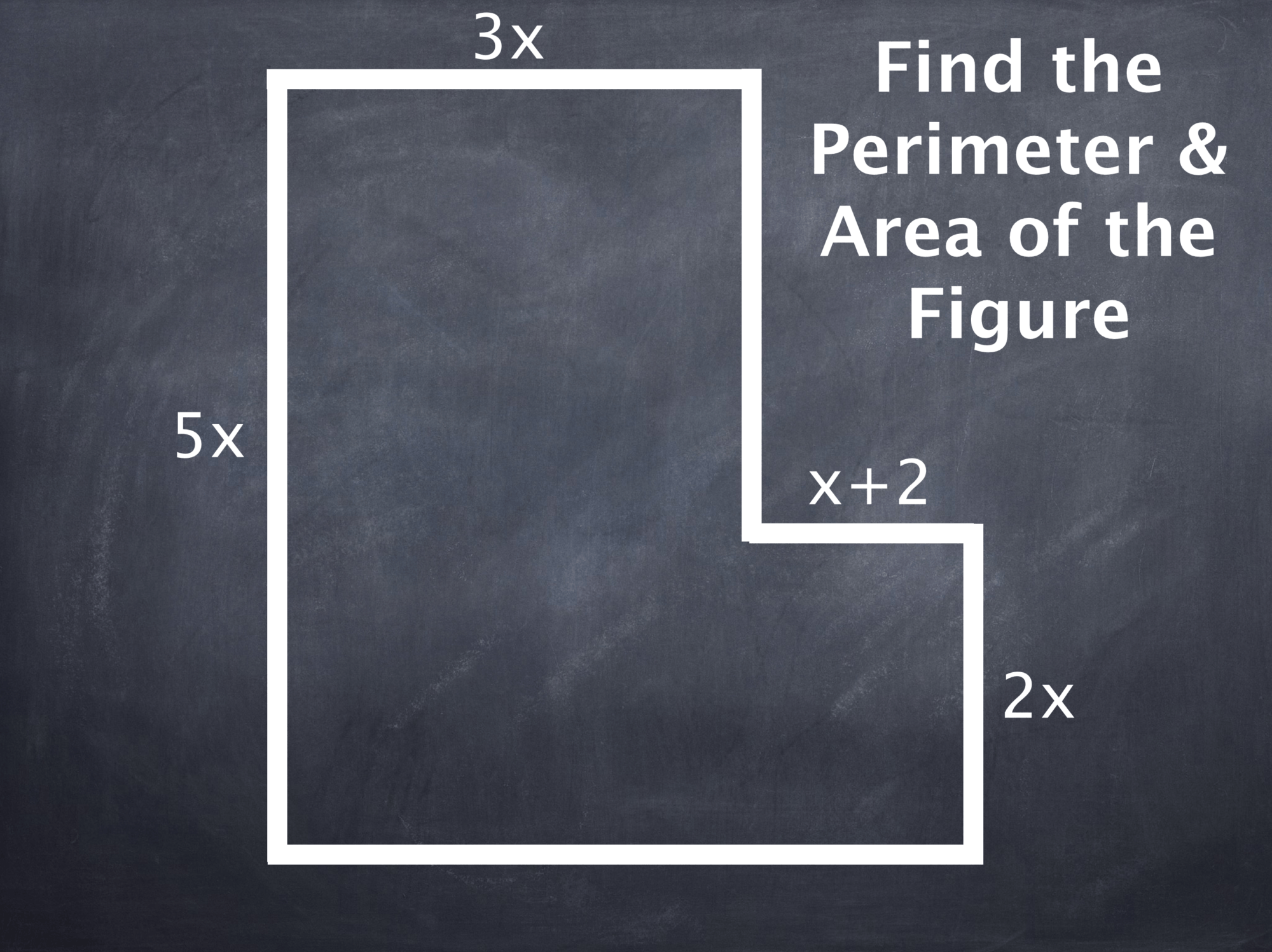In my experience, students can easily grasp the concept of finding the perimeter and area of a composite figure when all dimensions are known. However, when we begin introducing the same concept with an unknown value, students begin to show signs of anxiety. Eyes begin to gloss over, hands become clammy and beads of sweat begin to form on the forehead. Even if students begin to feel comfortable finding the perimeter and area of a simple shape like a rectangle with monomial term dimensions, introducing a binomial into the fold introduces new challenges: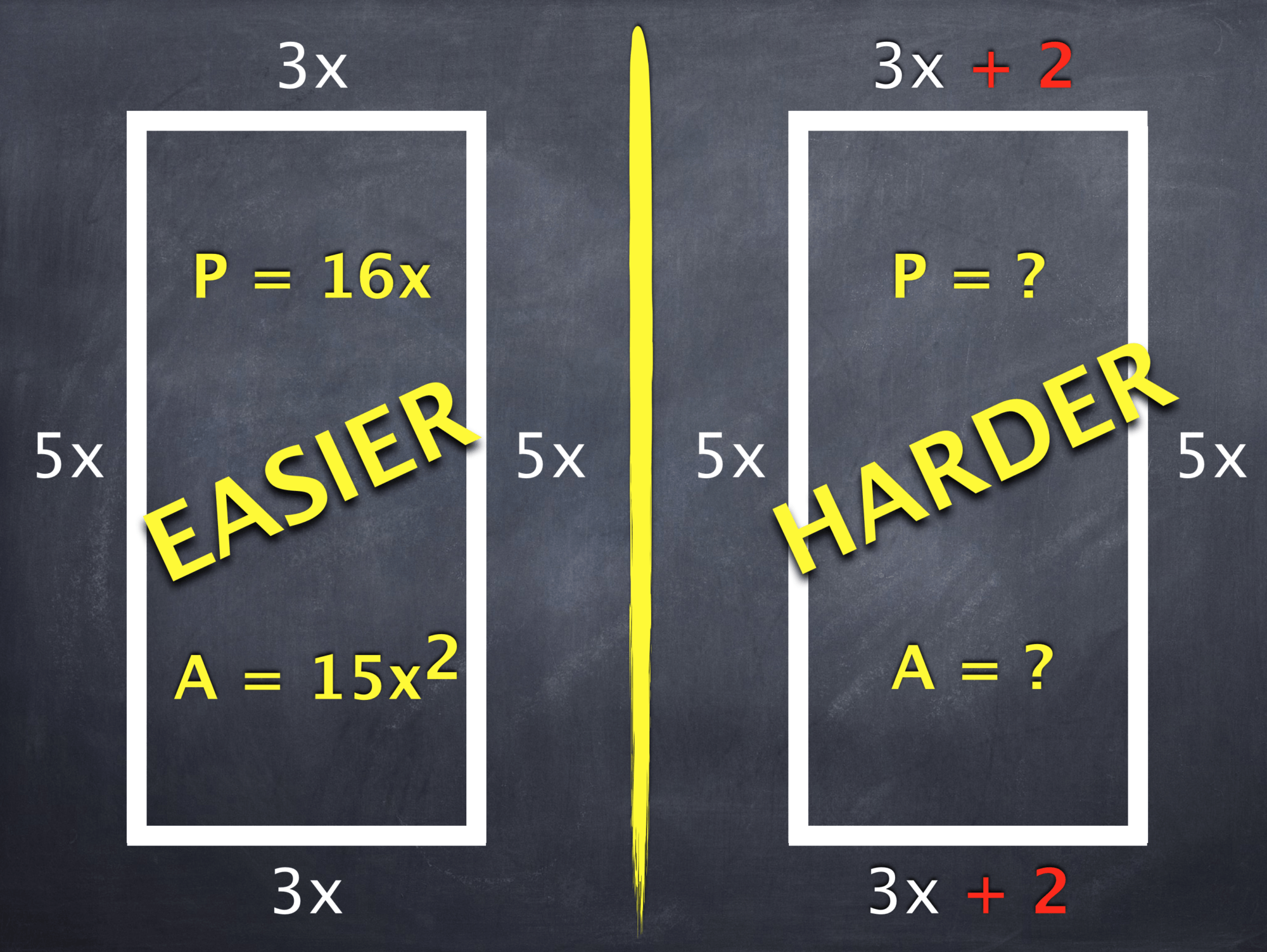## Concepts Related To The Problem:Shifting the focus back to our math textbook task redesign, the following concepts are related to the problem:

• Finding perimeter,
• finding area,
• applying perimeter and area to composite figures,
• simplifying expressions by collecting like terms,
• multiplying a first-degree monomial by a constant,
• multiplying a first-degree monomial by a first-degree monomial, and
• using distributive property to multiply a first-degree monomial by a first-degree binomial.

It’s no wonder our textbooks are written in such a way that each concept is isolated in a silo by itself and typically, students are to practice procedure repeatedly prior to introducing this type of problem.

However, if we think back to how we introduce the concept of multiplication through an array, we can use this prior knowledge as a springboard to provide students with an opportunity to apply their spatial reasoning skills and develop a deep understanding of what this problem really looks like.

## Visualizing Multiplication As An Array

Recalling a simple multiplication array model introduced in primary:we can then apply this concept to multiplying larger numbers by chunking them in an array model:Applying this same concept to the leftmost rectangle in our problem, we quickly note that the length is 5 times a number (5x), while the width is 3 times a number (3x). Let’s show the students what this looks like if we assume x is equal to 2.

### For Perimeter with x = 2:### For Area with x = 2: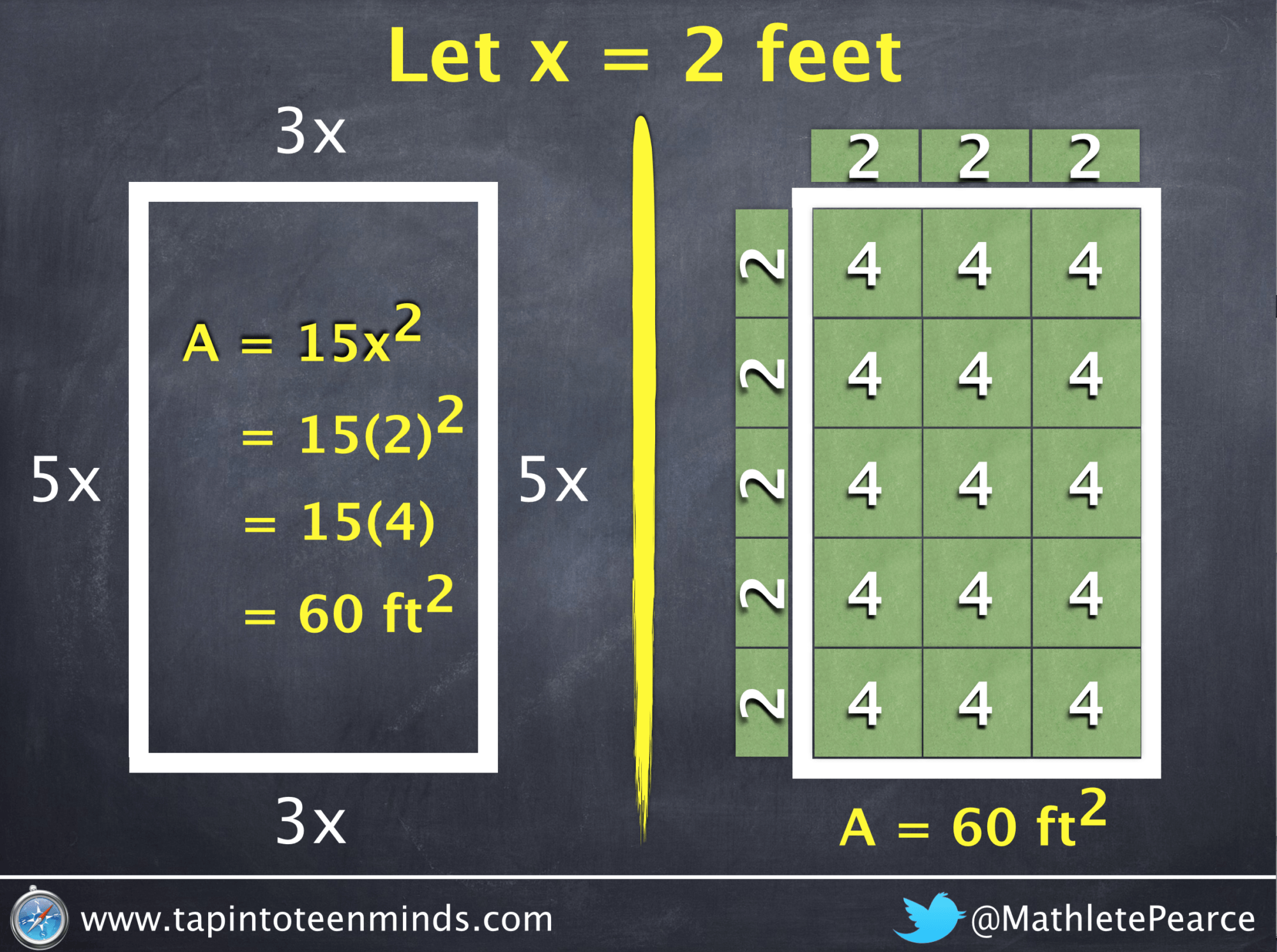You can see in the area example above that we can apply our chunking multiplication array model directly to a problem very similar to what we are working with here.

### For Perimeter with x unknown:

After introducing an unknown in place of the constant “2,” students should have an easier time determining “how many x’s there are” as you can see in this image below: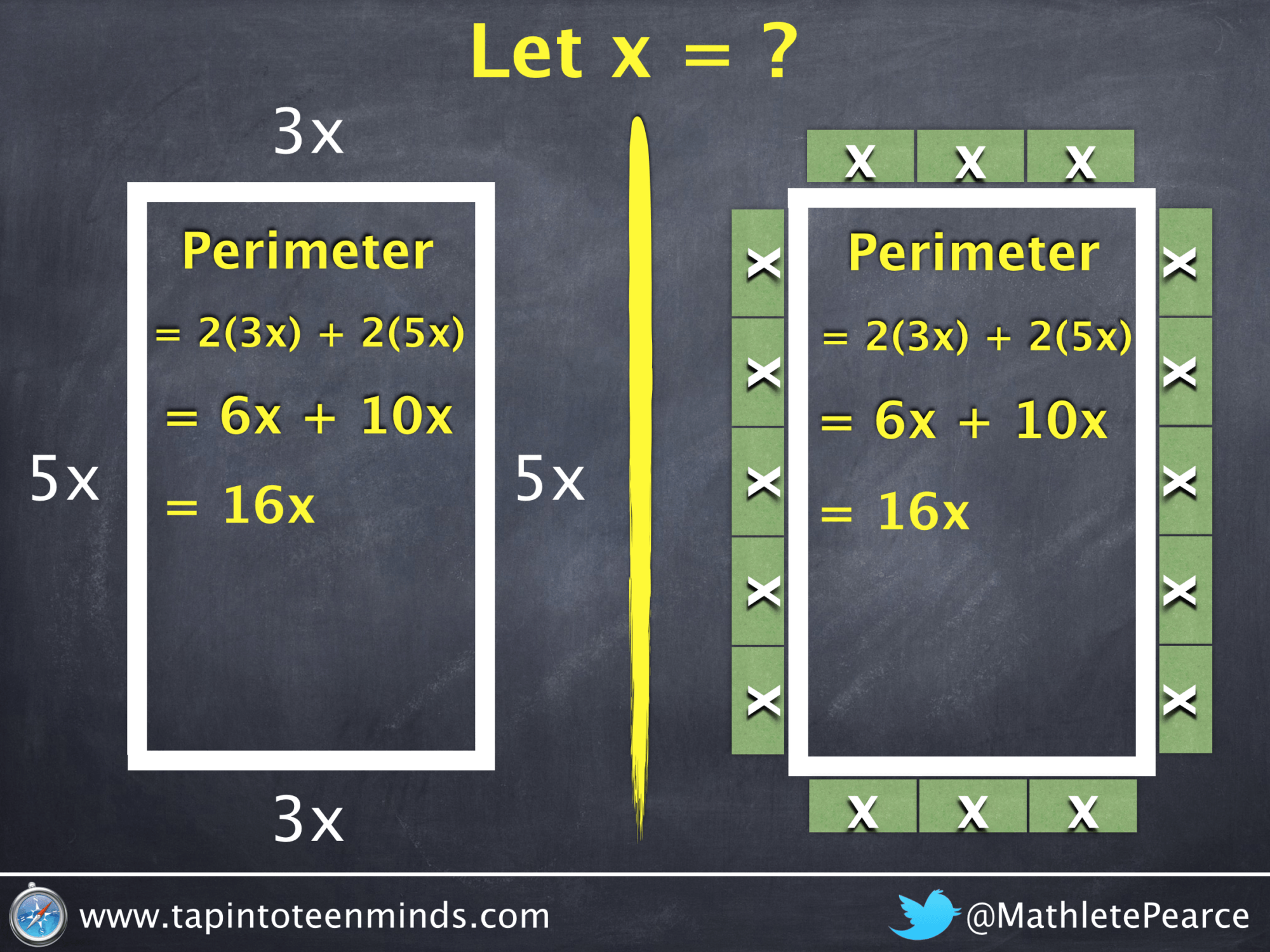### For Area with x unknown:

Now that we have applied our prior knowledge from primary when learning how to multiply, we can introduce an unknown without causing too much trouble due to the visualization provided: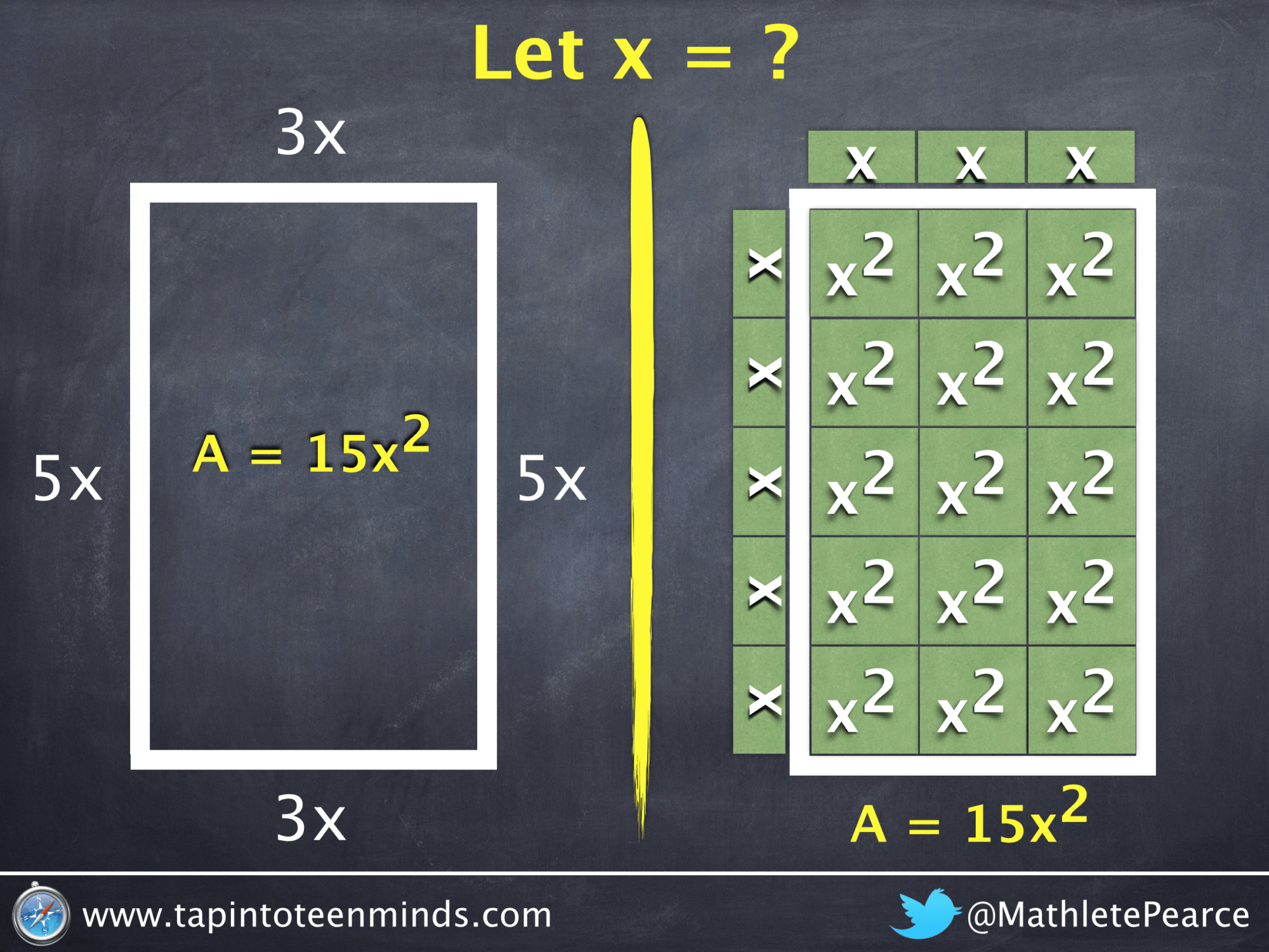## Math Task Redesign: Adding Context to the Problem

Now, we can take the spatial reasoning strategies we have introduced above and apply them to the same problem, but with some context added. In this case, students will be determining the perimeter and area of an L-Shaped Pool by using a pool noodle.

### Finding the Perimeter of the Pool

For perimeter, students can better visualize the distance around the pool when the following context is added:### Finding the Area of the Pool

For area, students may recall using grid paper to find area when in the junior grades to assist in their calculation. By using a pool noodle, students can quickly determine how many “pool noodles-squared” make up the area of the pool. Also note that we don’t even really need to formally discuss distribution at this point in time.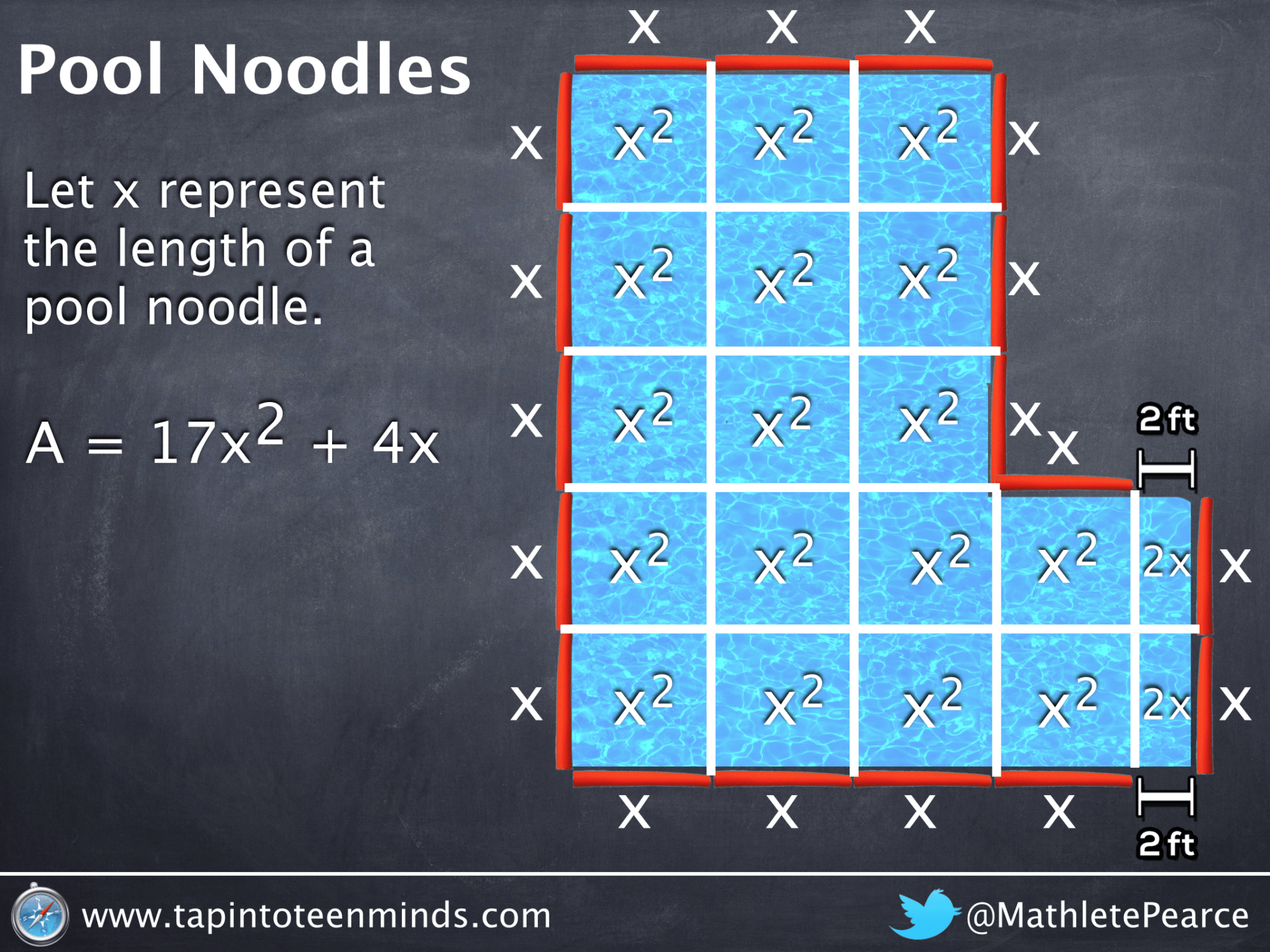### Consolidating the Learning: Finding the Area Using Distribution

When students are comfortable calculating the area visually as displayed in the image above, then and only then should we begin to have discussions about how we could do these calculations without “all the work.”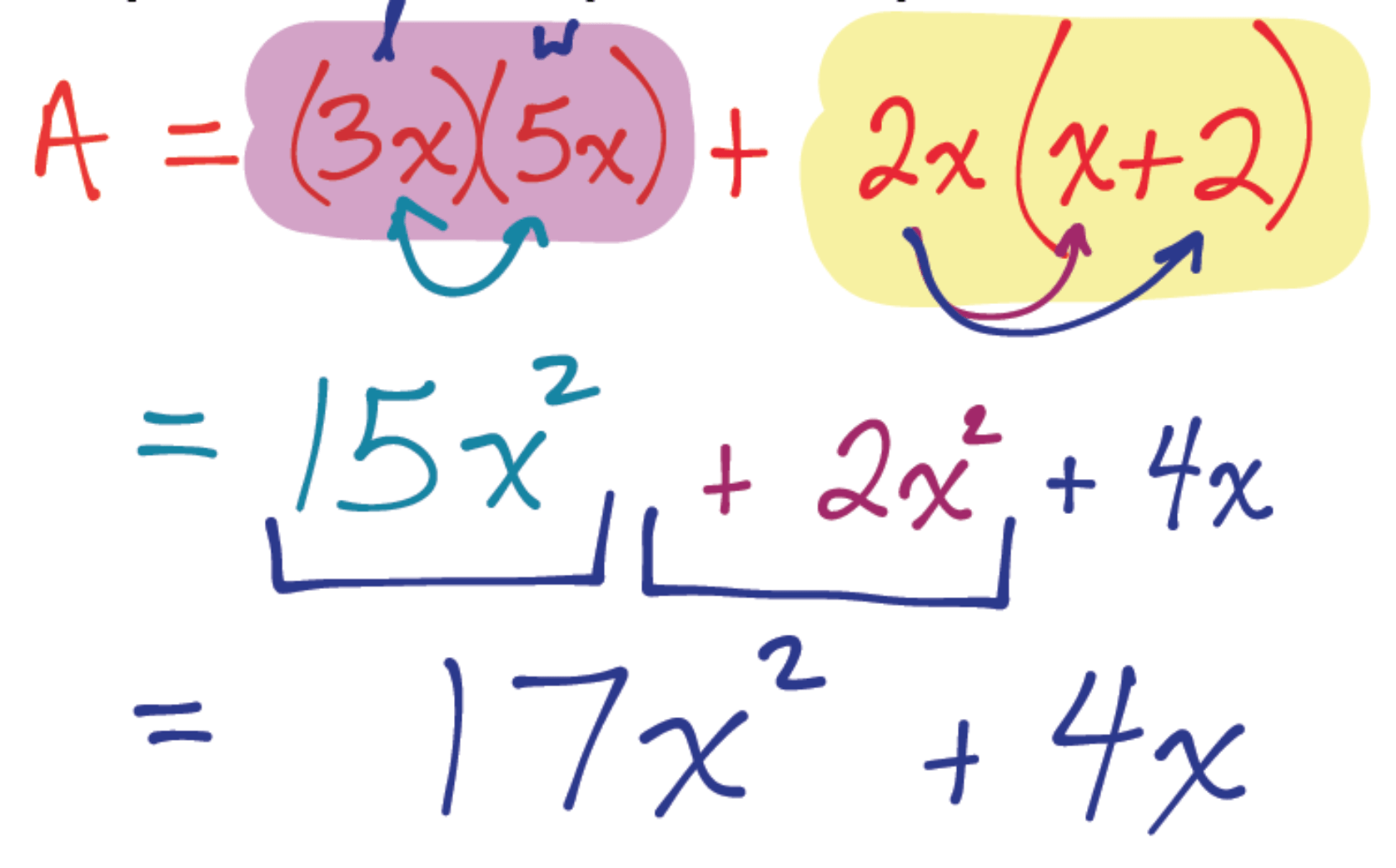If we allow students to develop and apply their spatial reasoning skills and apply them to mathematics daily, they will learn to appreciate algebra for what it was intended to do; make life easier! On the other hand, if we rush to the algebraic representation, not only will students not gain an appreciation for the algebraic representation, but they will likely find it much more difficult to understand due to the lack of spatial understanding.

I hope you try to apply the Pool Noodles Tapping It Up A Notch Task Redesign in your classroom. Let me know how it goes in the comments.

## Memorization vs. Automaticity

### What's The Difference?

Find out by downloading the 5-Page Printable Memorization vs. Automaticity Cheat Sheet to learn more! A ton of great learning to be had with this printable & sharable E-Book!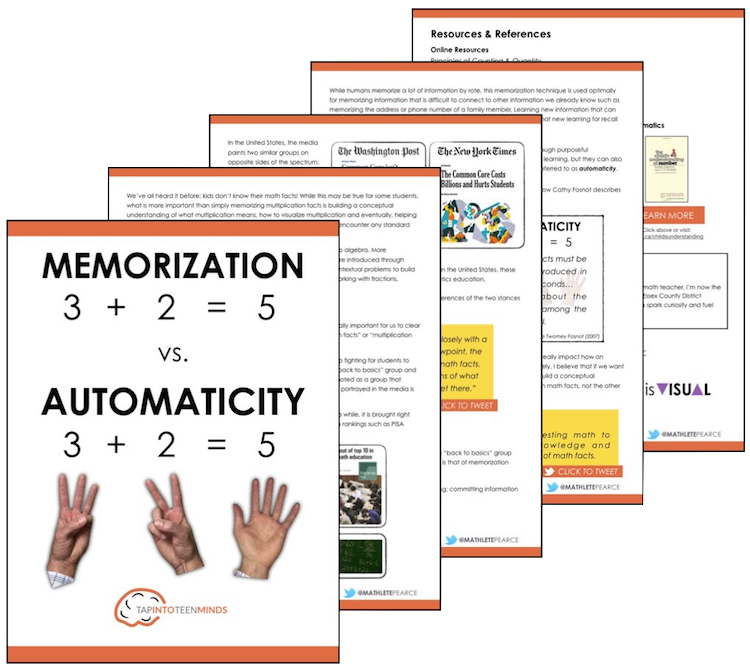## Share With Your Learning Community:## About Kyle Pearce

I’m Kyle Pearce and I am a former high school math teacher. I’m now the K-12 Mathematics Consultant with the Greater Essex County District School Board, where I uncover creative ways to spark curiosity and fuel sense making in mathematics. Read more.

Read More From The Blog

## Not What You're Looking For?

### Search By Criteria:

Subscribe